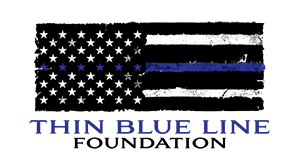# Back the Bluethis is sample content this is sample content this is sample content this is sample content this is sample content this is sample content this is sample content this is sample content this is sample content this is sample content this is sample content this is sample content this is sample content this is sample content this is sample content this is sample content this is sample content this is sample content this is sample content this is sample content this is sample content this is sample content this is sample content this is sample content this is sample content this is sample content

this is sample content this is sample content this is sample content this is sample content this is sample content this is sample content this is sample content this is sample content this is sample content this is sample content this is sample content this is sample content this is sample content this is sample content this is sample content this is sample content this is sample content this is sample content this is sample content this is sample content this is sample content this is sample content this is sample content this is sample content this is sample content this is sample content this is sample content this is sample content this is sample content this is sample content this is sample content this is sample content this is sample content this is sample content this is sample content this is sample content this is sample content this is sample content this is sample content this is sample content this is sample content this is sample content this is sample content this is sample content this is sample content this is sample content this is sample content